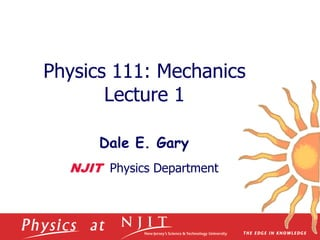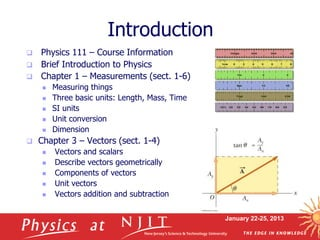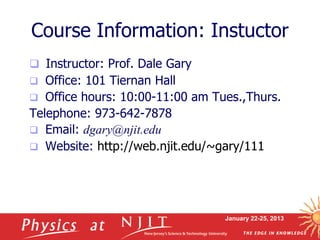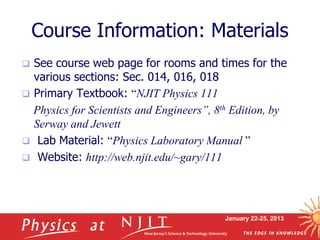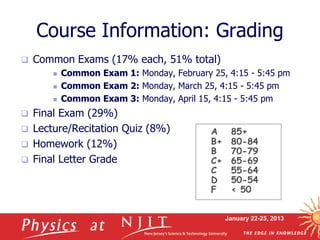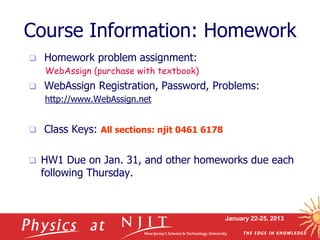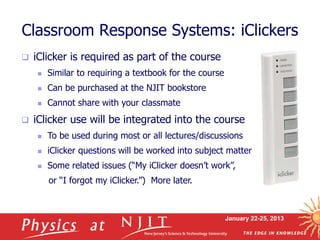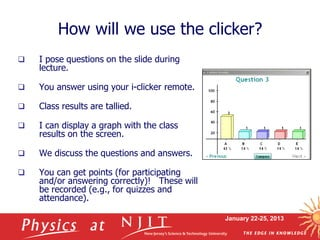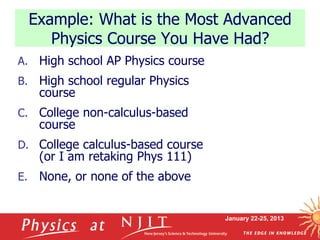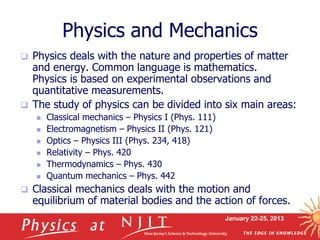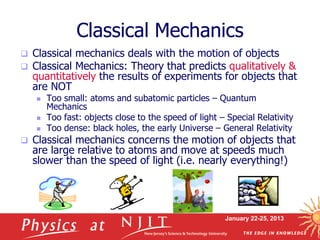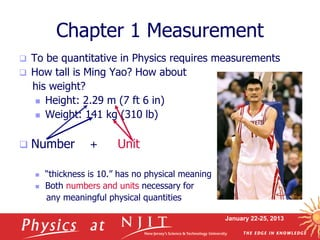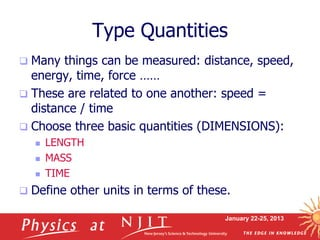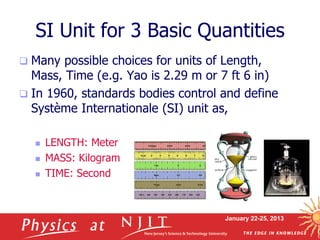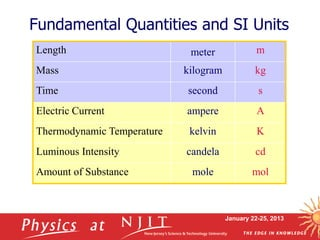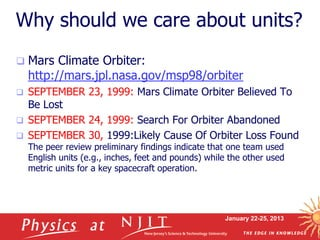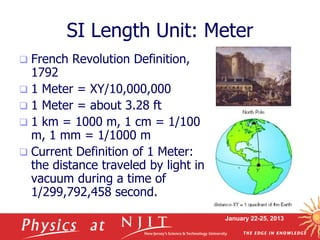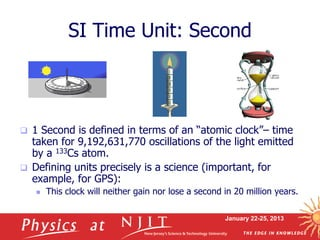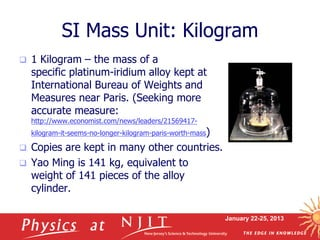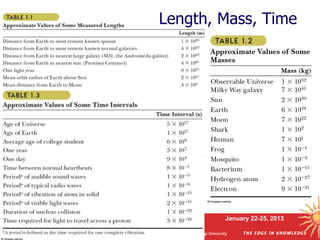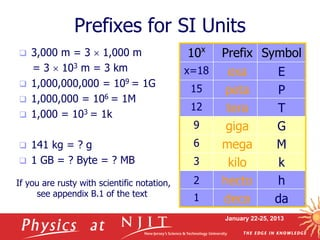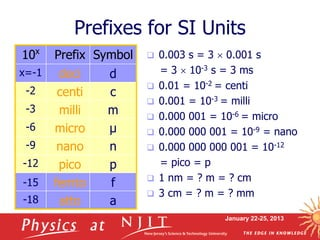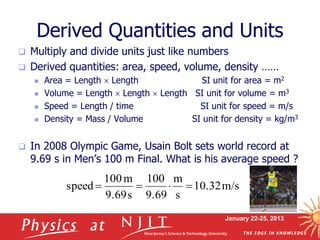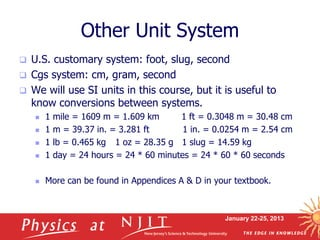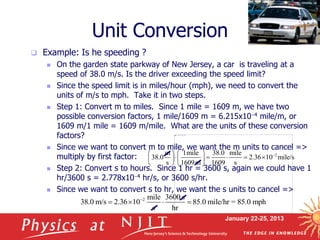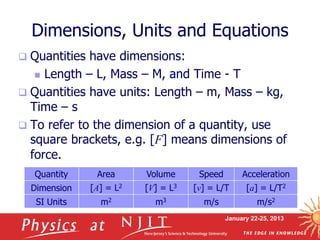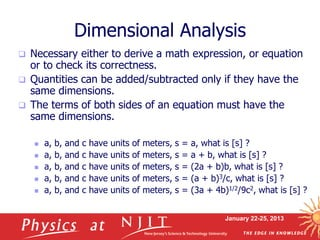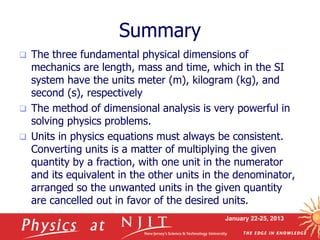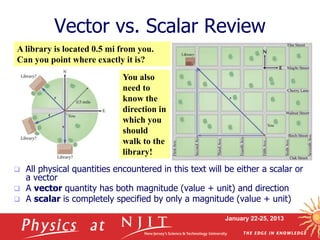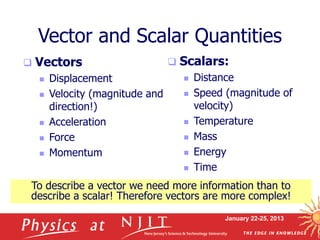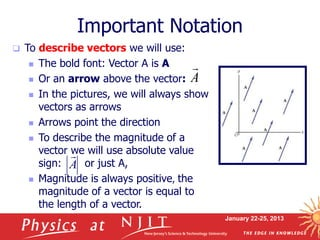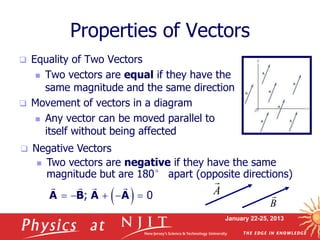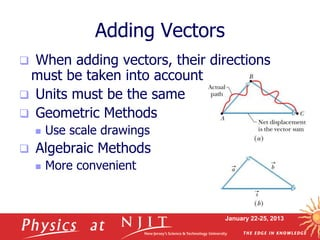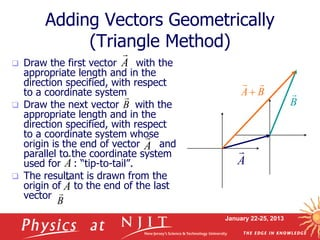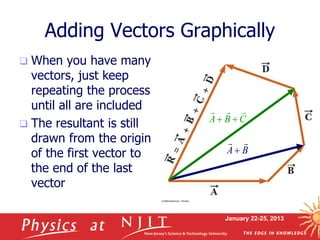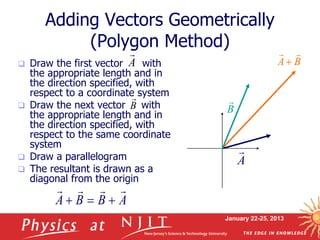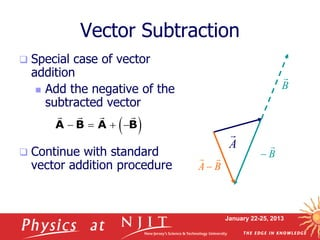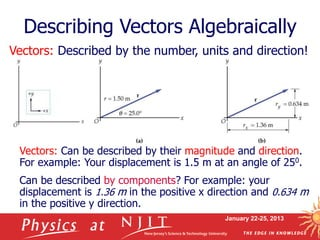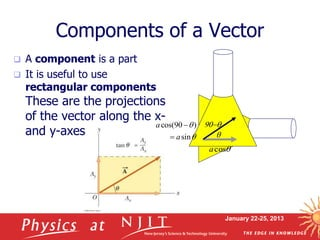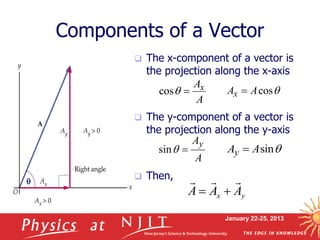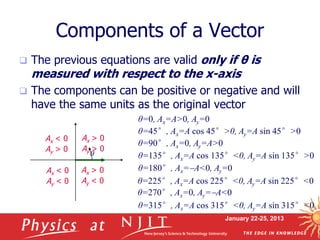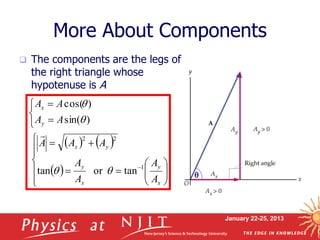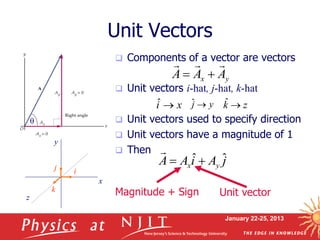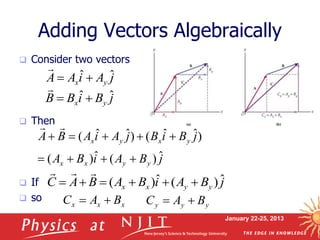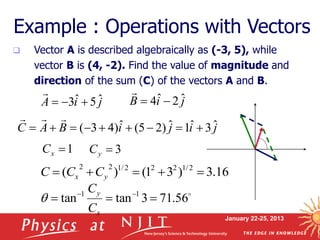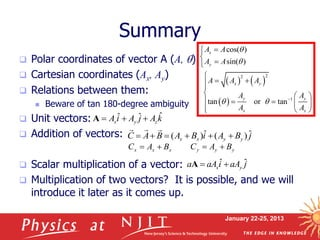1 of 46

### Phys111_lecture01.ppt

1. Physics 111: Mechanics Lecture 1 Dale E. Gary NJIT Physics Department
2. January 22-25, 2013 Introduction  Physics 111 – Course Information  Brief Introduction to Physics  Chapter 1 – Measurements (sect. 1-6)  Measuring things  Three basic units: Length, Mass, Time  SI units  Unit conversion  Dimension  Chapter 3 – Vectors (sect. 1-4)  Vectors and scalars  Describe vectors geometrically  Components of vectors  Unit vectors  Vectors addition and subtraction
3. January 22-25, 2013 Course Information: Instuctor  Instructor: Prof. Dale Gary  Office: 101 Tiernan Hall  Office hours: 10:00-11:00 am Tues.,Thurs. Telephone: 973-642-7878  Email: dgary@njit.edu  Website: http://web.njit.edu/~gary/111
4. January 22-25, 2013 Course Information: Materials  See course web page for rooms and times for the various sections: Sec. 014, 016, 018  Primary Textbook: “NJIT Physics 111 Physics for Scientists and Engineers”, 8th Edition, by Serway and Jewett  Lab Material: “Physics Laboratory Manual ”  Website: http://web.njit.edu/~gary/111
5. January 22-25, 2013 Course Information: Grading  Common Exams (17% each, 51% total)  Common Exam 1: Monday, February 25, 4:15 - 5:45 pm  Common Exam 2: Monday, March 25, 4:15 - 5:45 pm  Common Exam 3: Monday, April 15, 4:15 - 5:45 pm  Final Exam (29%)  Lecture/Recitation Quiz (8%)  Homework (12%)  Final Letter Grade A 85+ B+ 80-84 B 70-79 C+ 65-69 C 55-64 D 50-54 F < 50
6. January 22-25, 2013 Course Information: Homework  Homework problem assignment: WebAssign (purchase with textbook)  WebAssign Registration, Password, Problems: http://www.WebAssign.net  Class Keys: All sections: njit 0461 6178  HW1 Due on Jan. 31, and other homeworks due each following Thursday.
7. January 22-25, 2013 Classroom Response Systems: iClickers  iClicker is required as part of the course  Similar to requiring a textbook for the course  Can be purchased at the NJIT bookstore  Cannot share with your classmate  iClicker use will be integrated into the course  To be used during most or all lectures/discussions  iClicker questions will be worked into subject matter  Some related issues (“My iClicker doesn’t work”, or “I forgot my iClicker.”) More later.
8. January 22-25, 2013  I pose questions on the slide during lecture.  You answer using your i-clicker remote.  Class results are tallied.  I can display a graph with the class results on the screen.  We discuss the questions and answers.  You can get points (for participating and/or answering correctly)! These will be recorded (e.g., for quizzes and attendance). How will we use the clicker?
9. January 22-25, 2013 A. High school AP Physics course B. High school regular Physics course C. College non-calculus-based course D. College calculus-based course (or I am retaking Phys 111) E. None, or none of the above Example: What is the Most Advanced Physics Course You Have Had?
10. January 22-25, 2013 Physics and Mechanics  Physics deals with the nature and properties of matter and energy. Common language is mathematics. Physics is based on experimental observations and quantitative measurements.  The study of physics can be divided into six main areas:  Classical mechanics – Physics I (Phys. 111)  Electromagnetism – Physics II (Phys. 121)  Optics – Physics III (Phys. 234, 418)  Relativity – Phys. 420  Thermodynamics – Phys. 430  Quantum mechanics – Phys. 442  Classical mechanics deals with the motion and equilibrium of material bodies and the action of forces.
11. January 22-25, 2013 Classical Mechanics  Classical mechanics deals with the motion of objects  Classical Mechanics: Theory that predicts qualitatively & quantitatively the results of experiments for objects that are NOT  Too small: atoms and subatomic particles – Quantum Mechanics  Too fast: objects close to the speed of light – Special Relativity  Too dense: black holes, the early Universe – General Relativity  Classical mechanics concerns the motion of objects that are large relative to atoms and move at speeds much slower than the speed of light (i.e. nearly everything!)
12. January 22-25, 2013 Chapter 1 Measurement  To be quantitative in Physics requires measurements  How tall is Ming Yao? How about his weight?  Height: 2.29 m (7 ft 6 in)  Weight: 141 kg (310 lb)  Number + Unit  “thickness is 10.” has no physical meaning  Both numbers and units necessary for any meaningful physical quantities
13. January 22-25, 2013 Type Quantities  Many things can be measured: distance, speed, energy, time, force ……  These are related to one another: speed = distance / time  Choose three basic quantities (DIMENSIONS):  LENGTH  MASS  TIME  Define other units in terms of these.
14. January 22-25, 2013 SI Unit for 3 Basic Quantities  Many possible choices for units of Length, Mass, Time (e.g. Yao is 2.29 m or 7 ft 6 in)  In 1960, standards bodies control and define Système Internationale (SI) unit as,  LENGTH: Meter  MASS: Kilogram  TIME: Second
15. January 22-25, 2013 Fundamental Quantities and SI Units Length meter m Mass kilogram kg Time second s Electric Current ampere A Thermodynamic Temperature kelvin K Luminous Intensity candela cd Amount of Substance mole mol
16. January 22-25, 2013 Why should we care about units?  Mars Climate Orbiter: http://mars.jpl.nasa.gov/msp98/orbiter  SEPTEMBER 23, 1999: Mars Climate Orbiter Believed To Be Lost  SEPTEMBER 24, 1999: Search For Orbiter Abandoned  SEPTEMBER 30, 1999:Likely Cause Of Orbiter Loss Found The peer review preliminary findings indicate that one team used English units (e.g., inches, feet and pounds) while the other used metric units for a key spacecraft operation.
17. January 22-25, 2013 SI Length Unit: Meter  French Revolution Definition, 1792  1 Meter = XY/10,000,000  1 Meter = about 3.28 ft  1 km = 1000 m, 1 cm = 1/100 m, 1 mm = 1/1000 m  Current Definition of 1 Meter: the distance traveled by light in vacuum during a time of 1/299,792,458 second.
18. January 22-25, 2013 SI Time Unit: Second  1 Second is defined in terms of an “atomic clock”– time taken for 9,192,631,770 oscillations of the light emitted by a 133Cs atom.  Defining units precisely is a science (important, for example, for GPS):  This clock will neither gain nor lose a second in 20 million years.
19. January 22-25, 2013 SI Mass Unit: Kilogram  1 Kilogram – the mass of a specific platinum-iridium alloy kept at International Bureau of Weights and Measures near Paris. (Seeking more accurate measure: http://www.economist.com/news/leaders/21569417- kilogram-it-seems-no-longer-kilogram-paris-worth-mass)  Copies are kept in many other countries.  Yao Ming is 141 kg, equivalent to weight of 141 pieces of the alloy cylinder.
20. January 22-25, 2013 Length, Mass, Time
21. January 22-25, 2013 Prefixes for SI Units 10x Prefix Symbol x=18 exa E 15 peta P 12 tera T 9 giga G 6 mega M 3 kilo k 2 hecto h 1 deca da  3,000 m = 3  1,000 m = 3  103 m = 3 km  1,000,000,000 = 109 = 1G  1,000,000 = 106 = 1M  1,000 = 103 = 1k  141 kg = ? g  1 GB = ? Byte = ? MB If you are rusty with scientific notation, see appendix B.1 of the text
22. January 22-25, 2013 10x Prefix Symbol x=-1 deci d -2 centi c -3 milli m -6 micro µ -9 nano n -12 pico p -15 femto f -18 atto a Prefixes for SI Units  0.003 s = 3  0.001 s = 3  10-3 s = 3 ms  0.01 = 10-2 = centi  0.001 = 10-3 = milli  0.000 001 = 10-6 = micro  0.000 000 001 = 10-9 = nano  0.000 000 000 001 = 10-12 = pico = p  1 nm = ? m = ? cm  3 cm = ? m = ? mm
23. January 22-25, 2013 Derived Quantities and Units  Multiply and divide units just like numbers  Derived quantities: area, speed, volume, density ……  Area = Length  Length SI unit for area = m2  Volume = Length  Length  Length SI unit for volume = m3  Speed = Length / time SI unit for speed = m/s  Density = Mass / Volume SI unit for density = kg/m3  In 2008 Olympic Game, Usain Bolt sets world record at 9.69 s in Men’s 100 m Final. What is his average speed ? m/s 10.32 s m 9.69 100 s 9.69 m 100 speed    
24. January 22-25, 2013 Other Unit System  U.S. customary system: foot, slug, second  Cgs system: cm, gram, second  We will use SI units in this course, but it is useful to know conversions between systems.  1 mile = 1609 m = 1.609 km 1 ft = 0.3048 m = 30.48 cm  1 m = 39.37 in. = 3.281 ft 1 in. = 0.0254 m = 2.54 cm  1 lb = 0.465 kg 1 oz = 28.35 g 1 slug = 14.59 kg  1 day = 24 hours = 24 * 60 minutes = 24 * 60 * 60 seconds  More can be found in Appendices A & D in your textbook.
25. January 22-25, 2013 Unit Conversion  Example: Is he speeding ?  On the garden state parkway of New Jersey, a car is traveling at a speed of 38.0 m/s. Is the driver exceeding the speed limit?  Since the speed limit is in miles/hour (mph), we need to convert the units of m/s to mph. Take it in two steps.  Step 1: Convert m to miles. Since 1 mile = 1609 m, we have two possible conversion factors, 1 mile/1609 m = 6.215x10-4 mile/m, or 1609 m/1 mile = 1609 m/mile. What are the units of these conversion factors?  Since we want to convert m to mile, we want the m units to cancel => multiply by first factor:  Step 2: Convert s to hours. Since 1 hr = 3600 s, again we could have 1 hr/3600 s = 2.778x10-4 hr/s, or 3600 s/hr.  Since we want to convert s to hr, we want the s units to cancel => 2 m 1mile 38.0 mile 38.0 2.36 10 mile/s s 1609m 1609 s -                  2 mile 3600 s 38.0 m/s 2.36 10 85.0 mile/hr = 85.0 mph s hr -    
26. January 22-25, 2013  Quantities have dimensions:  Length – L, Mass – M, and Time - T  Quantities have units: Length – m, Mass – kg, Time – s  To refer to the dimension of a quantity, use square brackets, e.g. [F] means dimensions of force. Dimensions, Units and Equations Quantity Area Volume Speed Acceleration Dimension [A] = L2 [V] = L3 [v] = L/T [a] = L/T2 SI Units m2 m3 m/s m/s2
27. January 22-25, 2013 Dimensional Analysis  Necessary either to derive a math expression, or equation or to check its correctness.  Quantities can be added/subtracted only if they have the same dimensions.  The terms of both sides of an equation must have the same dimensions.  a, b, and c have units of meters, s = a, what is [s] ?  a, b, and c have units of meters, s = a + b, what is [s] ?  a, b, and c have units of meters, s = (2a + b)b, what is [s] ?  a, b, and c have units of meters, s = (a + b)3/c, what is [s] ?  a, b, and c have units of meters, s = (3a + 4b)1/2/9c2, what is [s] ?
28. January 22-25, 2013 Summary  The three fundamental physical dimensions of mechanics are length, mass and time, which in the SI system have the units meter (m), kilogram (kg), and second (s), respectively  The method of dimensional analysis is very powerful in solving physics problems.  Units in physics equations must always be consistent. Converting units is a matter of multiplying the given quantity by a fraction, with one unit in the numerator and its equivalent in the other units in the denominator, arranged so the unwanted units in the given quantity are cancelled out in favor of the desired units.
29. January 22-25, 2013 Vector vs. Scalar Review  All physical quantities encountered in this text will be either a scalar or a vector  A vector quantity has both magnitude (value + unit) and direction  A scalar is completely specified by only a magnitude (value + unit) A library is located 0.5 mi from you. Can you point where exactly it is? You also need to know the direction in which you should walk to the library!
30. January 22-25, 2013 Vector and Scalar Quantities  Vectors  Displacement  Velocity (magnitude and direction!)  Acceleration  Force  Momentum  Scalars:  Distance  Speed (magnitude of velocity)  Temperature  Mass  Energy  Time To describe a vector we need more information than to describe a scalar! Therefore vectors are more complex!
31. January 22-25, 2013 Important Notation  To describe vectors we will use:  The bold font: Vector A is A  Or an arrow above the vector:  In the pictures, we will always show vectors as arrows  Arrows point the direction  To describe the magnitude of a vector we will use absolute value sign: or just A,  Magnitude is always positive, the magnitude of a vector is equal to the length of a vector. A  A 
32. January 22-25, 2013 Properties of Vectors  Equality of Two Vectors  Two vectors are equal if they have the same magnitude and the same direction  Movement of vectors in a diagram  Any vector can be moved parallel to itself without being affected A   Negative Vectors  Two vectors are negative if they have the same magnitude but are 180° apart (opposite directions)   ; 0  -  -  A B A A B 
33. January 22-25, 2013 Adding Vectors  When adding vectors, their directions must be taken into account  Units must be the same  Geometric Methods  Use scale drawings  Algebraic Methods  More convenient
34. January 22-25, 2013 Adding Vectors Geometrically (Triangle Method)  Draw the first vector with the appropriate length and in the direction specified, with respect to a coordinate system  Draw the next vector with the appropriate length and in the direction specified, with respect to a coordinate system whose origin is the end of vector and parallel to the coordinate system used for : “tip-to-tail”.  The resultant is drawn from the origin of to the end of the last vector A  B  A  B A    A  B  A  A  B 
35. January 22-25, 2013 Adding Vectors Graphically  When you have many vectors, just keep repeating the process until all are included  The resultant is still drawn from the origin of the first vector to the end of the last vector B A    B A    C B A     
36. January 22-25, 2013 Adding Vectors Geometrically (Polygon Method)  Draw the first vector with the appropriate length and in the direction specified, with respect to a coordinate system  Draw the next vector with the appropriate length and in the direction specified, with respect to the same coordinate system  Draw a parallelogram  The resultant is drawn as a diagonal from the origin A  B  A  B A    B  A B B A       
37. January 22-25, 2013 Vector Subtraction  Special case of vector addition  Add the negative of the subtracted vector  Continue with standard vector addition procedure   -   - A B A B A  B  B A   - B  -
38. January 22-25, 2013 Describing Vectors Algebraically Vectors: Described by the number, units and direction! Vectors: Can be described by their magnitude and direction. For example: Your displacement is 1.5 m at an angle of 250. Can be described by components? For example: your displacement is 1.36 m in the positive x direction and 0.634 m in the positive y direction.
39. January 22-25, 2013 Components of a Vector  A component is a part  It is useful to use rectangular components These are the projections of the vector along the x- and y-axes q q cos a 90-q cos(90 ) sin a a q q - 
40. January 22-25, 2013 Components of a Vector  The x-component of a vector is the projection along the x-axis  The y-component of a vector is the projection along the y-axis  Then, x y   A A A q cos x A A q  cos x A A q  sin y A A q  sin y A A q  y x A A A     
41. January 22-25, 2013 Components of a Vector  The previous equations are valid only if θ is measured with respect to the x-axis  The components can be positive or negative and will have the same units as the original vector θ θ=0, Ax=A>0, Ay=0 θ=45°, Ax=A cos 45°>0, Ay=A sin 45°>0 θ=90°, Ax=0, Ay=A>0 θ=135°, Ax=A cos 135°<0, Ay=A sin 135°>0 θ=180°, Ax=-A<0, Ay=0 θ=225°, Ax=A cos 225°<0, Ay=A sin 225°<0 θ=270°, Ax=0, Ay=-A<0 θ=315°, Ax=A cos 315°<0, Ay=A sin 315°<0 Ax > 0 Ay > 0 Ax < 0 Ay > 0 Ax < 0 Ay < 0 Ax > 0 Ay < 0
42. January 22-25, 2013 More About Components  The components are the legs of the right triangle whose hypotenuse is A 2 2 1 tan y x y x A A A A and A q -                                        - x y x y y x y x A A A A A A A A A A A 1 2 2 tan or tan ) sin( ) cos( q q q q  Or, q
43. January 22-25, 2013 Unit Vectors  Components of a vector are vectors  Unit vectors i-hat, j-hat, k-hat  Unit vectors used to specify direction  Unit vectors have a magnitude of 1  Then x y   A A A q z k  ˆ x i  ˆ y j  ˆ y x z i j k y x A A A      j A i A A y x ˆ ˆ   Magnitude + Sign Unit vector
44. January 22-25, 2013 Adding Vectors Algebraically  Consider two vectors  Then  If  so x y   A A A j B A i B A B A C y y x x ˆ ) ( ˆ ) (          j B A i B A j B i B j A i A B A y y x x y x y x ˆ ) ( ˆ ) ( ) ˆ ˆ ( ) ˆ ˆ (            j B i B B y x ˆ ˆ   j A i A A y x ˆ ˆ   x x x B A C   y y y B A C  
45. January 22-25, 2013 Example : Operations with Vectors  Vector A is described algebraically as (-3, 5), while vector B is (4, -2). Find the value of magnitude and direction of the sum (C) of the vectors A and B. j i j i B A C ˆ 3 ˆ 1 ˆ ) 2 5 ( ˆ ) 4 3 (   -   -       j i B ˆ 2 ˆ 4 -   j i A ˆ 5 ˆ 3  -   1  x C 3  y C 16 . 3 ) 3 1 ( ) ( 2 / 1 2 2 2 / 1 2 2      y x C C C  56 . 71 3 tan tan 1 1    - - x y C C q
46. Summary  Polar coordinates of vector A (A, q)  Cartesian coordinates (Ax, Ay)  Relations between them:  Beware of tan 180-degree ambiguity  Unit vectors:  Addition of vectors:  Scalar multiplication of a vector:  Multiplication of two vectors? It is possible, and we will introduce it later as it comes up. January 22-25, 2013       2 2 1 cos( ) sin( ) tan or tan x y x y y y x x A A A A A A A A A A A q q q q -                         ˆ ˆ ˆ x y z A i A j A k    A j B A i B A B A C y y x x ˆ ) ( ˆ ) (          x x x B A C   y y y B A C   ˆ ˆ x y a aA i aA j   A